### Similarity And The Theorem Pythagoras Class 10th Mathematics Gujarat Board Solution

##### Question 1.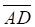,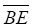,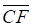are the medians of ΔABC. If BE = 12, CF = 9 and AB2 + BC2 + AC2 = 600, BC = 10, find AD.Answer:From the given info,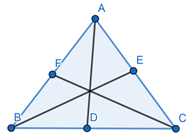As AB2 + BC2 + AC2 = 600and BC = 10,⇒ AB2 + AC2 = 500 ...... (1)As AD is median,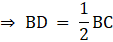⇒ BD = 5 ...... (2)In Δ ABC, using the theorem of Apolloneous, we know that,For AD to be the median, we have,AB2 + AC2 = 2(AD2 + BD2)Substituting values for (1) and (2) in above equation,⇒ 500 = 2 (AD2 + 52)⇒ 250 = AD2 + 25⇒ AD2 = 225⇒ AD2 = 152⇒ AD = 15Question 2.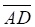is the altitude of Δ ABC such that B—D—C. If AD2 = BD ⋅ DC, prove that ∠BAC is right angle.Answer: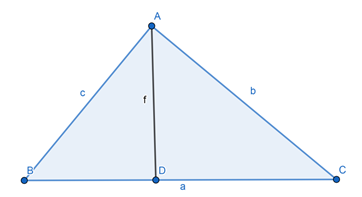Since AD is the altitude to BC,⇒ ∠BDA = ∠CDA = 90°So, Δ ABD and Δ ACD are right angle triangles.Using Pythagoras theorem,AB2 = BD2 + AD2 ...... (1)And, AC2 = AD2 + DC2 ...... (2)It is given that AD2 = BD.DC …… (3)From equation (1) and (3), we get,AB2 = BD2 + BD.DC⇒ AB2 = BD(BD + DC) = BD. BC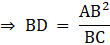Now, from equation (2) and (3),AC2 = BD.DC + DC2⇒ AC2 = DC (BD + DC)⇒ AC2 = DC(BC)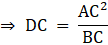Also, BC = BD + DC,Substituting the value of BD and DC in above equation, we get,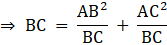⇒ BC2 = AB2 + AC2So, by the converse of Pythagoras Theorem, we can say that ∠BAC is a right angle.Question 3.In ΔABC,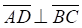, B—D—C. If AB2 = BD ⋅ BC, prove that ∠ BAC is a right angle.Answer: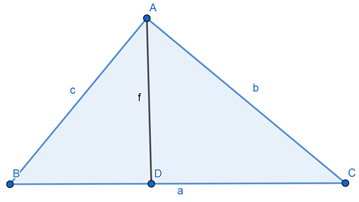Since AD is the altitude to BC,⇒ ∠BDA = ∠CDA = 90°So, Δ ABD and Δ ACD are right angle triangles.Using Pythagoras theorem,AB2 = BD2 + AD2 ...... (1)And, AC2 = AD2 + DC2 ...... (2)It is given that AB2 = BD.BCFrom equation (1) and (2), we get,AB2 – BD2 = AC2 – DC2⇒ AB2 – BD2 = AC2 – (BC – BD)2⇒ BC2 + BD2 – 2BC.BD = AC2 + BD2 – AB2⇒ BC2 – 2BC (BD) = AC2 – AB2Substituting the value of BD above, we get,⇒ BC2 – 2(AB)2 = AC2 – AB2⇒ BC2 = AB2 + AC2So, by the converse of Pythagoras Theorem, we can say that ∠BAC is a right angle.Question 4.In ΔABC,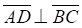, B—D—C. If AC2 = CD ⋅ BC, prove that ∠BAC is a right angle.Answer: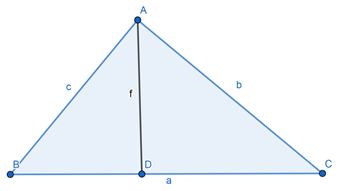Since AD is the altitude to BC,⇒ ∠BDA = ∠CDA = 90°So, Δ ABD and Δ ACD are right angle triangles.Using Pythagoras theorem,AB2 = BD2 + AD2 ...... (1)And, AC2 = AD2 + DC2 ...... (2)It is given that AC2 = CD.BC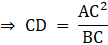From equation (1) and (2), we get,AB2 – BD2 = AC2 – CD2⇒ AB2 – (BC – CD)2 = AC2 – CD2⇒ AB2 – BC2 – CD2 + 2.BC.CD = AC2 – CD2⇒ AB2 – BC2 + 2.BC.CD = AC2Substituting the value of CD above, we get,⇒ AB2 – BC2 + 2AC2 = AC2⇒ AB2 + AC2 = BC2So, by the converse of Pythagoras Theorem, we can say that ∠BAC is a right angle.Question 5.is a median of ΔABC. If BD = AD, prove that ∠A is a right angle in ΔABC.Answer: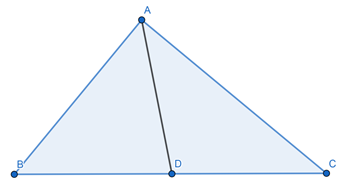In Δ ABC, using Apolloneous Theorem, we know that,For AD to be the median, we have,AB2 + AC2 = 2(AD2 + BD2)As it is given that AD = BD,⇒ AB2 + AC2 = 4.BD2Since AD is the median to line BC, we have,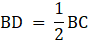Substituting the value of BD in above equation, we get,AB2 + AC2 = BC2So, by the converse of Pythagoras Theorem, we can say that ∠BAC is a right angle.Question 6.In figure 7.25, AC is the length of a pole standing vertical on the ground. The pole is bent at point B, so that the top of the pole touches the ground at a point 15 meters away from the base of the pole. If the length of the pole is 25, find the length of the upper part of the pole.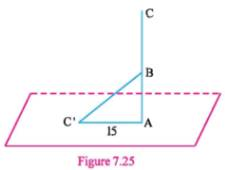Answer:Let the length of upper part of pole be x,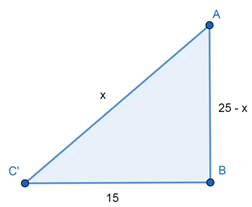The length of AB becomes 25 – x.Applying Pythagoras theorem in ΔABC,(AC’)2 = AB2 + (BC’)2⇒ x2 = (25 – x)2 + 152⇒ x2 = 625 + x2 – 50x + 225⇒ 50x = 850⇒ x = 17 m∴ The length of the upper part of the pole is 17 meters.Question 7.In ΔABC, AB > AC, D is the mid–point of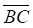.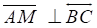such that B—M—C. Prove that AB2 — AC2 = 2BC . DM.Answer:From the given information, we construct the figure as: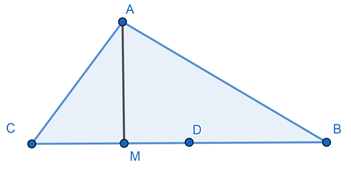As AM is perpendicular BC, ΔACM and ΔABM are right triangle.By using Pythagoras Theorem, we get,AC2 = CM2 + AM2 ...... (1)Also, AB2 = BM2 + AM2 ...... (2)Eliminating AM2 from equations (1) and (2), we get,AC2 – CM2 = AB2 – BM2⇒ AC2 – AB2 = CM2 – BM2By using the identity a2 – b2 = (a + b)(a – b) on above equation,⇒ AC2 – AB2 = (CM + BM)(CM – BM)⇒ AC2 – AB2 = BC(CM – (BC – CM))⇒ AB2 – AC2 = BC(2CM – BC)As D is mid point of BC,⇒ AB2 – AC2 = BC(2CM – 2BD)⇒ AB2 – AC2 = 2BC(CM – BD)⇒ AB2 – AC2 = 2BC(CM – CD)⇒ AB2 – AC2 = 2BC(–DM)⇒ AB2 — AC2 = 2BC.DMHence, proved.Question 8.In ΔABC,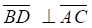, D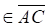and ∠B is right angle. If AC = 5CD, prove that BD = 2CD.Answer: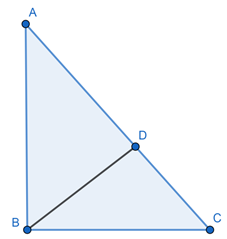ΔABC is a right triangle, right angle at B and BD is perpendicular from B vertex to hypotenuse AC.So, as we know that,If an altitude is drawn to hypotenuse of a right angled triangle, then the length of altitude is the geometric mean of lengths of segments of hypotenuse formed by the altitude.⇒ BD2 = AD.CD⇒ BD2 = (AC – CD).CDAs, AC = 5CD,⇒ BD2 = (5CD – CD).CD⇒ BD2 = 4CD2⇒ BD2 = (2CD)2⇒ BD = 2CDHence, proved.Question 9.In ΔPQR, if m∠P + m∠Q = m∠R. PR = 7, QR = 24, then PQ =A. 31B. 25C. 17D. 15Answer: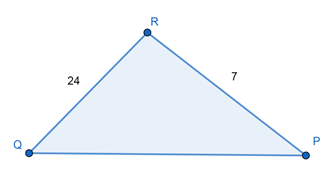In ΔABC, we know that sum of all interior angles in triangle is equal to 180°⇒ ∠P + ∠Q + ∠R = 180Also, given that, ∠P + ∠Q = ∠R,⇒ 2∠R = 180⇒ ∠R = 90°Using Pythagoras Theorem,PQ2 = PR2 + QR2⇒ PQ2 = 72 + (24)2⇒ PQ2 = 49 + 576⇒ PQ2 = 625⇒ PQ = 25∴ Option (b) is correct.Question 10.In ΔABC,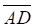is an altitude and ∠A is right angle. If AB =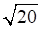, BD = 4, then CD =A. 5B. 3C. √5D. 1Answer: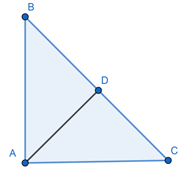As we know that,If an altitude is drawn to hypotenuse of a right angled triangle, then the length of each side other than the hypotenuse is the geometric mean of length of hypotenuse and segment of hypotenuse adjacent to the side.⇒ AB2 = BD.BC⇒ 20 = 4. BC⇒ BC = 5Also, CD = BC – BDCD = 5 – 4CD = 1∴ Option (d) is correct.Question 11.In ΔABC, AB2 + AC2 = 50. The length of the median AD = 3. So, BC =A. 4B. 24C. 8D. 16Answer: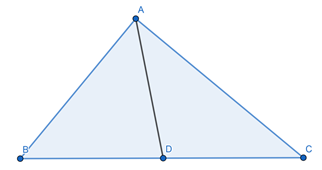In Δ ABC, using the theorem of Apolloneous, we know that,For AD to be the median, we have,AB2 + AC2 = 2(AD2 + BD2)⇒ 50 = 2(32 + BD2)⇒ 50 = 2 (9 + BD2)⇒ 25 = 9 + BD2⇒ BD2 = 16⇒ BD = 4⇒ BC = 2BD = 8∴ Option (c) is correct.Question 12.In ΔABC, m∠B = 90, AB = BC. Then AB: AC =A. 1:3B. 1:2C. 1 : √2D. √2:1Answer: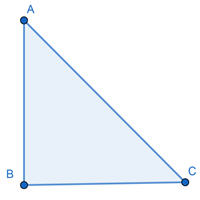In ΔABC, applying Pythagoras Theorem, we get,AC2 = AB2 + BC2As AB = BC,⇒ AC2 = 2AB2⇒ AB:AC = 1:√2∴ Option (c) is correct.Question 13.In ΔABC, m∠B = 90 and AC = 10. The length of the median BM =A. 5B. 5√2C. 6D. 8Answer: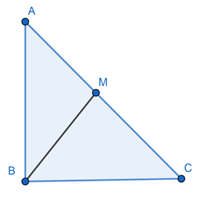In Δ ABC, using the theorem of Apolloneous, we know that,For BM to be the median, we have,AB2 + AC2 = 2(BM2 + CM2)Also, as Δ ABC is right angled at B,⇒ AB2 + AC2 = AC2 = 102 = 100⇒ 100 = 2 (BM2 + 52)⇒ 50 = BM2 + 25⇒ BM = 5∴ Option (a) is correct.Question 14.In ΔABC, AB = BC =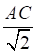. m∠BA. Is acuteB. Is obtuseC. Is right angleD. Cannot be obtainedAnswer: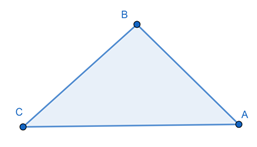Let us assume,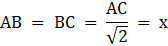⇒ AB = BC = x and AC = √2xAs we see that, AC2 = AB2 + BC2So by converse of Pythagoras Theorem, ΔABC is right angled and right angle at B.∴ Option (c) is correct.Question 15.In ΔABC, if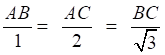then m ∠C = ……A. 90B. 30C. 60D. 45Answer: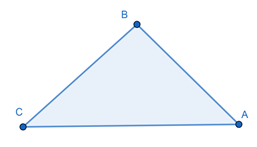Let us assume,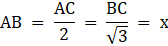⇒ AB = x, AC = 2x and BC = √3xAs we see that, AC2 = AB2 + BC2So by converse of Pythagoras Theorem, ΔABC is right angled and right angle at B.Let ∠C = θ,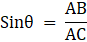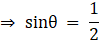⇒ θ = 30°∴ Option (b) is correct.Question 16.In ΔXYZ, m∠X : m∠Y : m∠Z = 1 : 2 : 3. If XY = 15, YZ = …A.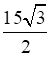B. 17C. 8D. 7.5Answer: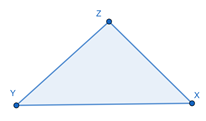Let ∠X = A,⇒ ∠Y = 2A and ∠Z = 3AAs sum of all angles in a triangle is equal to 180°∠X + ∠Y + ∠Z = 180⇒ 6A = 180⇒ A = 30⇒ ∠Z = 90°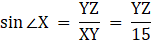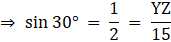⇒ YZ = 7.5∴ Option (d) is correct.Question 17.In Δ ABC, ∠B is a right angle and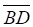is an altitude. If AD = BD = 5, then DC =A. 1B. √5C. 5D. 2.5Answer: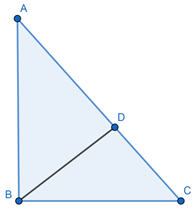As we know that,If an altitude is drawn to hypotenuse of a right angled triangle, then the length of altitude is the geometric mean of lengths of segments of hypotenuse formed by the altitude.BD2 = AD.CD⇒ 52 = 5.CD⇒ CD = 5∴ Option (c) is correct.Question 18.In Δ ABC,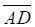is median. If AB2 + AC2 = 130 and AD = 7, then BD =A. 4B. 8C. 16D. 32Answer: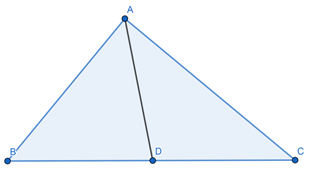In Δ ABC, using Apolloneous Theorem, we know that,For AD to be the median, we have,AB2 + AC2 = 2(AD2 + BD2)⇒ 130 = 2(72 + BD2)⇒ 65 = 49 + BD2⇒ 16 = BD2⇒ BD = 4∴ Option (a) is correct.Question 19.The diagonal of a square is 5√2. The length of the side of the square isA. 10B. 5C. 3√2D. 2√2Answer: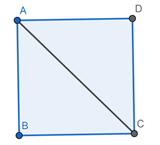Let the side of square be a.Diagonal = √2a⇒ 5√2 = √2a⇒ a = 5∴ Option (b) is correct.Question 20.The length of a diagonal of a rectangle is 13. If one of the side of the rectangle is 5, the perimeter of the rectangle is …A. 36B. 34C. 48D. 52Answer: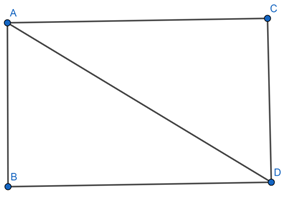Let the other side of rectangle be xBy Pythagoras Theorem,AD2 = AC2 + CD2132 = AC2 + 52⇒ 169 – 25 = AC2⇒ 144 = AC2⇒ AC2 = 122⇒ AC = 12Perimeter = 2(AC + CD)⇒ Perimeter = 2(12 + 5)⇒ Perimeter = 34∴ Option (b) is correct.Question 21.The length of a median of an equilateral triangle is √3. Length of the side of the triangle isA. 1B. 2√3C. 2D. √3Answer: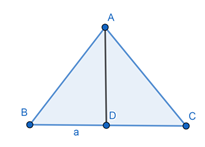Median of an equilateral triangle is also a perpendicular to the base.Let the side be a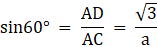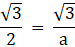⇒ a = 2∴ Option (c) is correct.Question 22.The perimeter of an equilateral triangle is 6. The length of the altitude of the triangle is …A. 4B. 2√3C. 2D. √3Answer:Let the length of altitude be x and side be a.⇒ 3a = 6⇒ a = 2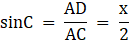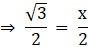⇒ x = √3∴ Option (d) is correct.Question 23.In ΔABC, m∠A = 90.is a median. If AD = 6, AB = 10, then AC =A. 8B. 7.5C. 16D. 2√11Answer: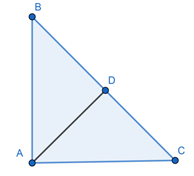In Δ ABC, using Apolloneous Theorem, we know that,For AD to be the median, we have,AB2 + AC2 = 2(AD2 + BD2)⇒ BC2 = 2 (36 + BD2)⇒ 4BD2 = 72 + 2BD2⇒ 2BD2 = 72⇒ BD2 = 36⇒ BD = 6So, BC = 12 as BC = 2BDBy Pythagoras Theorem in ΔABC,AC2 = BC2 – AB2⇒ AC2 = 144 – 100⇒ AC = 2√11∴ Option (d) is correct.Question 24.In Δ PQR, m∠Q = 90 and PQ = QR.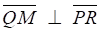,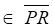. If QM = 2, PQ =A. 4B. 2√2C. 8D. 2Answer: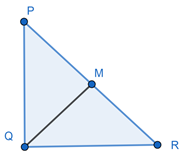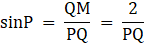Since ∠Q = 90 and PQ = QR , therefore ∠QPR = ∠QRP = θAs sum of all of triangle = 180°⇒ 2θ + 90 = 180⇒ θ = 45°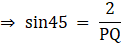⇒ PQ = 2√2∴ Option (b) is correct.Question 25.In Δ ABC, m∠A = 90,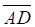is an altitude. So AB2 = ……A. BD.BCB. BD.DCC.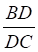D. BC.DCAnswer:By Pythagoras Theorem in ΔABD,AB2 = AD2 + BD2 ...... (1)As we know that,If an altitude is drawn to hypotenuse of a right angled triangle, then the length of altitude is the geometric mean of lengths of segments of hypotenuse formed by the altitude.AD2 = BD.CD ...... (2)From equation (1) and (2)AB2 = BD.CD + BD2⇒ AB2 = BD(CD + BD)⇒ AB2 = BD.BC∴ Option (a) is correct.Question 26.In Δ ABC, m∠A = 90,is an altitude. Therefore BD.DC = ……A. AB2B. BC2C. AC2D. AD2Answer:As we know that,If an altitude is drawn to hypotenuse of a right angled triangle, then the length of altitude is the geometric mean of lengths of segments of hypotenuse formed by the altitude.⇒ AD2 = BD.DC∴ Option (d) is correct.

PDF FILE TO YOUR EMAIL IMMEDIATELY PURCHASE NOTES & PAPER SOLUTION. @ Rs. 50/- each (GST extra)

HINDI ENTIRE PAPER SOLUTION

MARATHI PAPER SOLUTION

SSC MATHS I PAPER SOLUTION

SSC MATHS II PAPER SOLUTION

SSC SCIENCE I PAPER SOLUTION

SSC SCIENCE II PAPER SOLUTION

SSC ENGLISH PAPER SOLUTION

SSC & HSC ENGLISH WRITING SKILL

HSC ACCOUNTS NOTES

HSC OCM NOTES

HSC ECONOMICS NOTES

HSC SECRETARIAL PRACTICE NOTES

# 2019 Board Paper Solution

HSC ENGLISH SET A 2019 21st February, 2019

HSC ENGLISH SET B 2019 21st February, 2019

HSC ENGLISH SET C 2019 21st February, 2019

HSC ENGLISH SET D 2019 21st February, 2019

SECRETARIAL PRACTICE (S.P) 2019 25th February, 2019

HSC XII PHYSICS 2019 25th February, 2019

CHEMISTRY XII HSC SOLUTION 27th, February, 2019

OCM PAPER SOLUTION 2019 27th, February, 2019

HSC MATHS PAPER SOLUTION COMMERCE, 2nd March, 2019

HSC MATHS PAPER SOLUTION SCIENCE 2nd, March, 2019

SSC ENGLISH STD 10 5TH MARCH, 2019.

HSC XII ACCOUNTS 2019 6th March, 2019

HSC XII BIOLOGY 2019 6TH March, 2019

HSC XII ECONOMICS 9Th March 2019

SSC Maths I March 2019 Solution 10th Standard11th, March, 2019

SSC MATHS II MARCH 2019 SOLUTION 10TH STD.13th March, 2019

SSC SCIENCE I MARCH 2019 SOLUTION 10TH STD. 15th March, 2019.

SSC SCIENCE II MARCH 2019 SOLUTION 10TH STD. 18th March, 2019.

SSC SOCIAL SCIENCE I MARCH 2019 SOLUTION20th March, 2019

SSC SOCIAL SCIENCE II MARCH 2019 SOLUTION, 22nd March, 2019

XII CBSE - BOARD - MARCH - 2019 ENGLISH - QP + SOLUTIONS, 2nd March, 2019

# HSCMaharashtraBoardPapers2020

(Std 12th English Medium)

HSC ECONOMICS MARCH 2020

HSC OCM MARCH 2020

HSC ACCOUNTS MARCH 2020

HSC S.P. MARCH 2020

HSC ENGLISH MARCH 2020

HSC HINDI MARCH 2020

HSC MARATHI MARCH 2020

HSC MATHS MARCH 2020

# SSCMaharashtraBoardPapers2020

(Std 10th English Medium)

English MARCH 2020

HindI MARCH 2020

Hindi (Composite) MARCH 2020

Marathi MARCH 2020

Mathematics (Paper 1) MARCH 2020

Mathematics (Paper 2) MARCH 2020

Sanskrit MARCH 2020

Sanskrit (Composite) MARCH 2020

Science (Paper 1) MARCH 2020

Science (Paper 2)

Geography Model Set 1 2020-2021

MUST REMEMBER THINGS on the day of Exam

Are you prepared? for English Grammar in Board Exam.

Paper Presentation In Board Exam

How to Score Good Marks in SSC Board Exams

Tips To Score More Than 90% Marks In 12th Board Exam

How to write English exams?

How to prepare for board exam when less time is left

How to memorise what you learn for board exam

No. 1 Simple Hack, you can try out, in preparing for Board Exam

How to Study for CBSE Class 10 Board Exams Subject Wise Tips?

JEE Main 2020 Registration Process – Exam Pattern & Important Dates

NEET UG 2020 Registration Process Exam Pattern & Important Dates

How can One Prepare for two Competitive Exams at the same time?

8 Proven Tips to Handle Anxiety before Exams!